# Correlation ratio

(diff) ← Older revision | Latest revision (diff) | Newer revision → (diff)
A characteristic of dependence between random variables. The correlation ratio of a random variablerelative to a random variableis the expression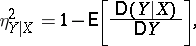whereis the variance of,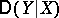is the conditional variance ofgiven, which characterizes the spread ofabout its conditional mathematical expectation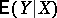for a given value of. Invariably,. The equality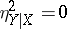corresponds to non-correlated random variables;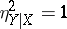if and only if there is an exact functional relationship betweenand; ifis linearly dependent on, the correlation ratio coincides with the squared correlation coefficient. The correlation ratio is non-symmetric inand, and so, together with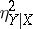, one considers(the correlation ratio ofrelative to, defined analogously). There is no simple relationship betweenand. See also Correlation (in statistics).